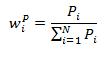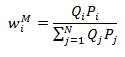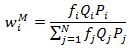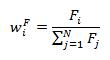### Why should I choose AnalystNotes?

Simply put: AnalystNotes offers the best value and the best product available to help you pass your exams.

##### Subject 2. Index Construction and Management

The steps to construct and manage a security market index:

• The first decision is to identify the target market. Which market should the index represent?

• The second decision is to select specific securities to include in the index. How many securities to include? Which ones? The following factors are important:

• Size: the larger, the better - but eventually the costs of taking a larger sample will outweigh the benefits.
• The breadth of the sample: the sample must represent the total population.
• The source of the sample: samples must be taken from each different segment of the population.

• The third decision is to determine the weight to be allocated to each security in the index (discussed below).

• When should the index be rebalanced?

• When should the security selection and weighting decisions be re-examined?

Price Weighting

This is an arithmetic average of current prices. Index movements are influenced by the differential prices of the components.

The weight of each security is calculated using this formula:The index itself is computed by:

• Adding up the market price of each stock in the index, then
• Dividing this total price by the number of stocks in the index: price-weighted series = sum of stock prices / number of stocks in the series.

Example

Shares of firm A sell for \$100 and shares of firm B sell for \$25. The initial price index is (100 + 25) / 2 = 62.5. The divisor is therefore 2.

• Normal situation. Suppose that A increases by 10% to \$110 and B increases by 20% to \$30; the price index would be (110 + 30) /2 = 70. The rate of return would be: (70 - 62.5) / 62.5 = 12%.

• Stock split. If A were to split two for one, and its share price were therefore to fall to \$50, we would not want the average to fall since that would incorrectly indicate a fall in the general level of market prices. Following a split, the divisor must be reduced to a value that leaves the average unaffected by the split. The new divisor is: (50 + 25) / 62.5 = 1.2, which will make the initial value of the average unaffected.

Price-weighting is simple, but a price-weighted index has a downward bias.

• High-priced stocks have a greater impact on the index than low-priced stocks, as the scheme assumes that an investor purchases an equal number of shares for each stock in the index.

• Large successful firms consistently lose weight within the index since high-growth companies tend to split their stocks more often. Over time, low-growth small firms with high prices will dominate the index.

Both the Dow Jones Industrial Average (DJIA) and the Nikkei-Dow Jones Average use this method to weight an index.

Equal Weighting

All stocks carry equal weight regardless of their price or market value. A \$1 stock is as important as a \$10 stock, and a firm with a \$200 million market value is the same as one with a \$200 billion value.

The actual movements in the index are typically based on the arithmetic average of the percent changes in price or value for the stocks in the index: each percent change has equal weight. Such an index can be used by individuals who randomly select stock for their portfolios and invest the same dollar amount in each stock.

The weight of each security is calculated using this formula:It assumes that equal dollar amounts are invested in each stock in the index at the beginning of the period. It is typically generated by taking the arithmetic or geometric mean of the percentage changes in the value of the stocks in the index.

The primary advantage of equal weighting is its simplicity. However, since the prices of securities keep changing, the index needs to be rebalanced frequently to maintain equal weights.

Market-Capitalization Weighting

This measurement is generated by deriving the initial total market value of all stocks used in the series. The importance of individual stocks in the sample depends on the market value of the stocks. There is an automatic adjustment for stock splits and other capital changes in this series.

The weight of each security is calculated using this formula:Qi is the number of shares outstanding of security i.

A market-value-weighted series is generated by:

• Adding up the total market value of all stocks in the index: market value = number of shares outstanding x current market value.

• Dividing this total by the total market value for the base period.

• Multiplying this ratio by the beginning index value: new market value = (current market value / base value) x beginning index value.

Example

Shares of firm A sell for \$100 with 1 million shares and shares of firm B sell for \$25 with 20 million shares. Their market value is therefore \$100 million and \$500 million, respectively. If A increases by 10% to \$110 and B increases by 20% to \$30, their market value will be \$110 million and \$600 million, respectively. The rate of return would be: (710 - 600) / 600 = 18.3%.

As you can see, firms with large market value have greater impact on the index than firms with small market value. Thus, over time the large-market-value stocks will dominate changes in a market-value-weighted series.

A free-float adjustment factor is introduced in the float-adjusted market-capitalization weighting. It represents the proportion of shares that are free-floated as a percentage of issued shares. The index therefore does not include restricted stocks.Fundamental Weighting

Fundamentally based indices are indices in which stocks are weighted by one of many economic fundamental factors, especially accounting figures, which are commonly used when performing corporate valuation, or by a composite of several fundamental factors.Index Management: Rebalancing and Reconstitution

Rebalancing is adjusting the weights of the constituent securities in an index. This is done to maintain the weight of each security consistent with the index's weighting method. There is no need to rebalance price-weighted indices.

Companies may disappear through mergers or acquisitions, or they can become insolvent. A company may no longer satisfy the requirements for index inclusion. Changing the composition of an index is called reconstitution. Reconstitution is undertaken to ensure the index represents the desired target market.

Rebalancing and reconstitution create turnover in an index. Reconstitution can dramatically affect the prices of current and prospective constituents.

User Comment
arendb Do any disadvantages for Fundamental weighting exist?
johntan1979 How and why do you rebalance an equal-weighted index when it is EQUALLY WEIGHTED in the first place, regardless of price, market cap?

weightage = 1/N where N = number of different firms' securities
Naoual if you invest \$100 in an equal-weighted index constituted of 2 securities, you will by \$50 of each. As price changes, lets say to: \$60 and \$45, total=105; weights: 60/105=57% and 43% => your index is no longer equally weighted.
dirdeb @johntan1979 Market cap depends on the price of the constituents, as price changes so will the weighting of specific stocks. It needs rebalancing to maintain the equal weights withing the index.
Natk @CFAToad Stock splits don't affect index values - as in the example above, you adjust the constituents for splits.
mioa128 A bit confused about the equal-weighting methods. The formula indicates that the weight assigned to each security is independent of the price of the security. If that's the case, why does it need rebalancing?
mcbreatz The initial weight is just 1/number of securities. for example 5 securities 1/5=.2 or 20%. This is multiplied by total invested say \$1000. You should spend \$200 buying each security. After 1 period some securities will have performed better than others meaning they may make up more than .2 of the total value say 1100. Now each security should have a total of \$220 and adjustments will probably be needed. Typed way more than I though I would need when I started explaining that.